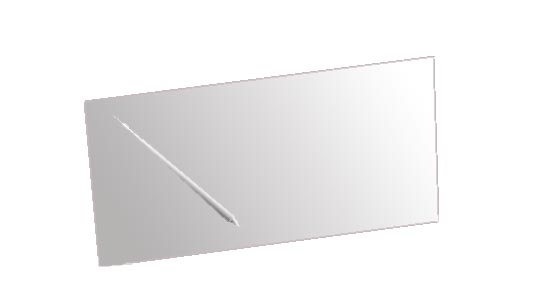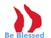The Silver Tablet

Home

PART ONE

The Challenge

The DNA Program

Computer Basics

DNA Code.
Program Coding

Simple Statistics

Why Statistics?

Self Created Comper VIRUS ?

Genius's Defence

A Mindless Programmer

Energy?

A bginning?

Impossible?

The jungle

Something Out Of Nothing?

Summary

Now The Real Challenge

7nbsp

PART TWO

PART TREE

Blogs

# A comparison between Binary Code and DNA COding.

There are many remarkable similarities between the DNA code and the binary code used by computers, but there are also important differences

First the simalarities:

1 Both codes are just copies (software) and need a hardware environment to execute a program (to do something that the prgram intends them to do).
2. It is easy to copy or to make copies of the code if the correct tools are available.
3 Both are able to assimilate foreign code.
4 Both codes are influenced by other programs and can communicate with other programs

Lets compare these simmalarities in more detail

1.Both are just copies.

We will illustrate this principle with a simple example. We will imagine that program that is able to generate wriiten language is coded in both types of code. The program will locate letters of the Western ( Roman) alphabet and arrange them in a predetermined way. The letters are either arrange in a logic way or in a chaotic way.

The DNA coding the example

Three bases in the DNA code , represent a letter in our example. Abbreviation are used.
Cytosine = C
Guanine = G
Thymine = U
Uracil (the base in RNA) = U

Our code representing the letters of the alphabet
AAA = a
CCC = b
GGG = c
TTT = d
ACG = e
GCA = f
AAC = g
CGG = h
ACC = i
CAA = j
AGT = k
ACT = l
CGT = m
TGA = n
TCG = o
TTG = p
AAG = q
AGG = r
GAA = s
GGA = t
CCT = u
CTT = v
TTC = w
TCC = x
GGC = y
GCC = z
TCA = br> letter that follows this code is in upper case
GCA = space

The DNA code coding the next sentence look like this:

GGACGGACCGAAGCAACCGAAGCAAAAGCATTCACGCCCGCATTGAAAAACACG

In our Western alphabeth the sentence will look this:

this is a web page

The string that contains the code in the DNA molecule will seperate from its partner and reduplicate the code onto a RNA molecule. Different RNA molecules will than arrange the letters to spell this is a web page.

Refer to THIS PAGE : DNA Program . The DNA code is discussed in more detail here.

The Binary ( Computer code) code coding for the example program. Binary computer code contains only two variables. An electronic circut is either open or closed. THe computer only counts to 2 ( our decimal system count to ten) Decimal 0, 1, 2. 3, 4, 5, 6, 7, 8 or 9 Binary 0 or 1. 11001100 = a
11000100 = b
11000110 = c
11001000 = d
11001010 = e
11001100 = f
11001110 = g
11010000 = h
11010010 = i
11010100 = j
11010110 = k
11011000 = l
11011010 = m
11011100 = n
11011110 = o
11100000 = p
11100010 = q
11100100 = r
11100110 = s
11101000 = t
11101010 = u
11101100 = v
11101110 = w
11110000 = x
11110010 = y
11110100 = z
11010000 = space

The following code represent the following sentencde

this is a web page

1110100011010000110100101110011011100110110100101110011011100110110011001101000011101110110010101100010011010000 11100000110011001100111011001010

Code not given here will transfer the sample code to the computers CPU ( central processing unit) and the CPU will send the code to the screen and the following message will appear on the screen

this is a web page

Do you agree? Genetic and binary code are simmalar. The argument that the genetic code is just another coding system like other codng systems is not valid. All other coding systems are the fruit of human intelligence. The genetic code was developed by a mindles mother nature with zero intelligence. ztrue?

2. It is easy to copy or to make copies of the code if the correct tools are available.
the second simalarity.